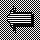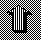# Feature Combination

For completeness, we should note that an alternative to selecting a subset of features is to combine the features to generate a smaller but more effective feature set.* The classic procedure is to form linear combinations, so that if x is the original d-dimensional feature vector and W is an d-by-m matrix, then the new m-dimensional feature vector y is given by

y = W' x .

The problem is to find the matrix W.

A frequently proposed "solution" to this problem is to use principal components analysis, or PCA. Here one forms the covariance matrix C for the example feature vectors, and finds the eigenvalues and eigenvectors of C. The m eigenvectors having the largest eigenvalues are then used as the columns of W. **

PCA can be shown to be optimal in a least-squares sense for representing the example feature vectors. Unfortunately, it usually does not provide the best linear combination of features for discriminating between the different classes. A more appropriate alternative is to use multiple discriminant analysis or MDA (see Duda and Hart). A full exposition of MDA is beyond the scope of these notes. However, the two-class case is simple. In this case, it turns out that W is the d-by-1 vector w given by

w = C-1 (m1 - m2) ,

where m1 is the mean vector for Class 1 and m2 is the mean vector for Class 2. The resulting single feature y = w' x is often called Fisher's linear discriminant. Unfortunately, MDA does not provide additional features if this feature turns out to be inadequate.

__________
* In essence, this is what the use of a "hidden layer" accomplishes in feedforward neural networks. Feature combination is also sometimes called feature extraction, the idea being that the combination draws together or "extracts" the meaningful features from the primitive features.

** A more modern approach is to perform a singular-value decomposition of the matrix of example feature vectors. However, this alternative suffers from the same shortcomings as classical PCA.Back to StepwiseUp to Feature Selection